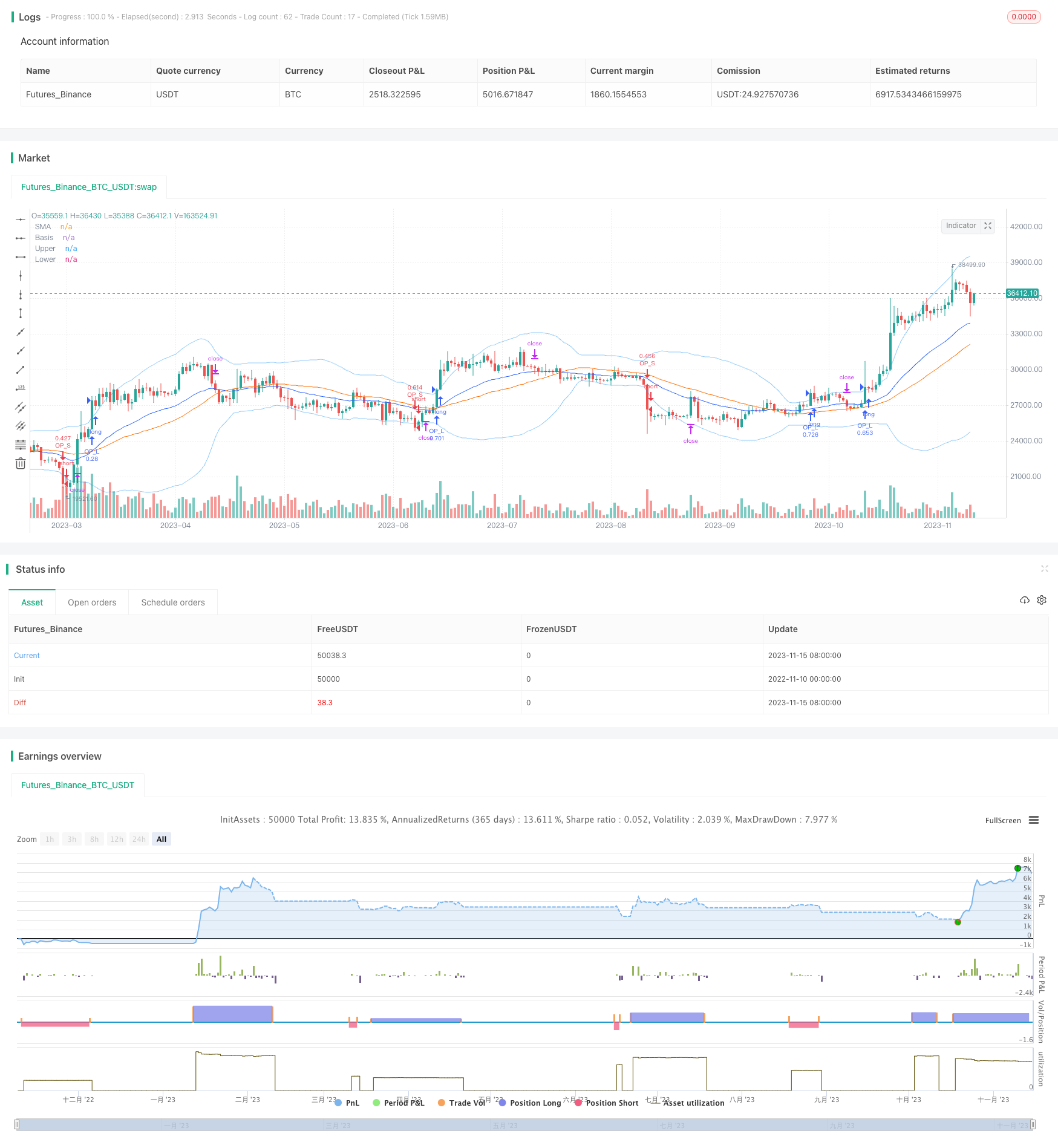# Trend Tracking Strategy Based on Bollinger Bands and Exponential Moving Average

Author: ChaoZhang, Date: 2023-11-17 17:36:43
Tags:## Overview

This strategy uses Bollinger Bands to determine the current trend direction and exponential moving average for stop loss and take profit management to effectively capture the trend.

## Principles

The strategy first calculates the middle line, upper band and lower band of Bollinger Bands. The middle line is the simple moving average of the closing price over n days. The upper and lower bands are shifted up and down by two standard deviations from the middle line. When the closing price is above the upper band, it indicates an uptrend. When the closing price is below the lower band, it indicates a downtrend.

The strategy judges the current trend direction by comparing the relationship between the closing price and the upper/lower bands of Bollinger Bands. If the closing price breaks through the upper band, go long. If the closing price breaks through the lower band, go short.

In addition, the exponential moving average is introduced as a trailing stop for stop loss and take profit. Specifically, if the price moves down after going long, the stop loss line will move down accordingly, gradually tightening the stop loss distance to maximize profit locking. If the price keeps rising, the stop loss line will also move up to let the profit run. The stop loss mechanism works in reverse for short positions.

The strategy combining Bollinger Bands for trend direction and EMA for stop loss/take profit management has the following advantages:

1. Using Bollinger Bands can effectively determine the trend direction and react quickly to breakouts.

2. EMA-based stop loss/take profit can maximize profit locking while controlling risks.

3. The strategy has few parameters which are easy to implement - just one for BB and one for EMA, very simple.

4. It can be widely applied to different products with strong adaptability.

## Risks and Optimization

The strategy also has some risks to note:

1. Breaking through BB upper/lower bands cannot completely avoid the risk of false breakouts. Consider combining with volume etc. to filter signals.

2. EMA parameter setting needs careful testing according to specific products. Too short EMA period may increase stop loss times. Too long will decrease trailing effectiveness.

3. Over-optimization needs to be avoided. Too many combinations of BB and EMA parameters may lead to overfitting.

For addressing the risks and optimization directions, the following can be considered:

1. Add volume or MACD etc. to filter false breakout signals.

2. Optimize EMA period through testing to find the most suitable parameter for specific products.

3. Try to keep BB and EMA parameters stable as much as possible to avoid overfitting risks from over-optimization.

4. Consider using RSI etc. to determine position adjustment in the mid-term trend.

## Summary

This strategy integrates using Bollinger Bands to determine the trend and EMA for stop loss/take profit management to form a relatively complete trend tracking system. It can quickly capture the trend direction and lock in profits by continuously adjusting the stop loss line. Overall, the strategy is relatively simple, practical and adaptable, worth further testing and optimization. But the parameter settings and risk control need to be noted to prevent misjudgments and over-optimization. Combining with other technical indicators for further improvement will be the direction going forward.

```/*backtest
start: 2022-11-10 00:00:00
end: 2023-11-16 00:00:00
period: 1d
basePeriod: 1h
exchanges: [{"eid":"Futures_Binance","currency":"BTC_USDT"}]
*/

// This source code is subject to the terms of the Mozilla Public License 2.0 at https://mozilla.org/MPL/2.0/
//@version=4
strategy(shorttitle=" BB+EMA", title="Bollinger Bands", overlay=true)
date1 = input(title="Start Date", type=input.time, defval=timestamp("2020-01-01T00:00:00"))
date2 = input(title="Stop Date", type=input.time, defval=timestamp("2030-01-01T00:00:00"))
length = input(40, minval=1)
src = input(close, title="Source")
mult = input(2.0,title="StdDev",step=0.1)
basis = sma(src, length)
dev = mult * stdev(src, length)
upper = basis + dev
lower = basis - dev
//offset = input(0, "Offset", minval = -500, maxval = 500)
offset=0
stopcon=input(title="stopcon/lot", type=input.bool, defval=true)
lot1=input(title="lot",defval=1)
stoploss=input(title="stopcon",defval=1000)
emacon=input(title="emacon", type=input.bool, defval=true)
ema_value=input(title="value",defval=30, minval=2,step=1)
plot(basis, "Basis", color=#FF6D00, offset = offset)
p1 = plot(upper, "Upper", color=color.new(color.blue,50), offset = offset)
p2 = plot(lower, "Lower", color=color.new(color.blue,50), offset = offset)
ema1=ema(close,ema_value)
plot(ema1, "SMA", color=#2962FF)
period() => true
//-----------
if period()
if close>upper
lot_L=stoploss/((close-lower)/2)
strategy.entry("OP_L",strategy.long,qty=stopcon==true?lot_L:lot1,stop=emacon==true?max(basis,ema1):basis)